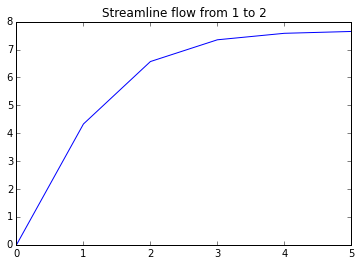# Chapter6-Incompressible Inviscid Flow¶

## Ex1-pg236¶

In :
import math
#calculate volume flow rate
##Depth of the duct(in m):
d=0.3;
##Width of the duct(in m):
w=0.1;
##Inner radius of the bend(in m):
r=0.25;
##Pressure difference between the taps(in mm of Hg):
p=40.;
##Density of water(in kg/m**3):
dw=999.;
##Acceleration due to gravity(in m/sec**2):
g=9.8;
##Density of air(in kg/m**3):
da=1.23;

##Volume flow rate##
##Velocity of flow(in m/sec):
V=math.sqrt(dw/math.log((r+w)/r)*g/da*p/1000.)
##Volume flow rate(in m**3/sec):
Q=V*(d*w)
print("RESULTS")
print'%s %.2f %s'%("Volume flow rate: ",Q," m^3/sec")

RESULTS
Volume flow rate:  0.92  m^3/sec


## Ex2-pg242¶

In :
import math
#calculate Velocity of flow
##Pressure diference(in mm of mecury):
p=30.;
##Density of water(in kg/m**3):
dw=1000.;
##Aceleration due to gravity(in m/sec**2):
g=9.81;
##Density of air(in kg/m**3):
da=1.23;
##Specific gravity of mercury:
SG=13.6;
##Velocity of flow##
##Velocity of flow(in m/sec):
V=math.sqrt(2.*dw*g*p/1000.*SG/da)
print("RESULTS")
print'%s %.2f %s'%("Velocity of flow: ",V," m/sec")

RESULTS
Velocity of flow:  80.67  m/sec


## Ex3-pg243¶

In :
##Area of nozzle at input(in m**2):
#calculate Gauge prssure required at the inlet
Ai=0.1;
##Area of nozzle at exit(in m**2):
Ae=0.02;
##Outlet velocity of flow(in m/sec):
V2=50;
##Density of air(in kg/m**3):
da=1.23;
#Velocity of flwat the inlet(in m/sec):
V1=Ae/Ai*V2
##Gauge pressure required at the inlet(in kPa):
p=0.5*da*(V2**2.-V1**2.)
print("RESULTS")
print'%s %.2f %s'%("Gauge prssure required at the inlet: ",p/1000," kPa")

RESULTS
Gauge prssure required at the inlet:  1.48  kPa


## Ex4-pg244¶

In :
#calculate Speed of water at exit:  and Pressure at point A in the flow
import math
##Length of tube above surface(in m):
l=1.;
##Depth of exit below water surface(in m):
z=7.;
##Acceleration due to gravity(in m/sec**2):
g=9.81;
##Density of water(in kg/m**3):
d=999.;
##Atmospheric pressure(in N/m**2):
p1=1.01*10**5;

##Speed and pressure##
##Speed of water at exit(in m/sec):
V2=math.sqrt(2.*g*z)
##Pressure at point A in the flow(kPa):
pA=p1+d*g*(0.-l)-0.5*d*V2**2.
print("RESULTS")
print'%s %.2f %s'%("Speed of water at exit: ",V2," m/sec")
print'%s %.2f %s'%("Pressure at point A in the flow: ",pA/1000," kPa")

RESULTS
Speed of water at exit:  11.72  m/sec
Pressure at point A in the flow:  22.60  kPa


## Ex5-pg246¶

In :
import math
#calculate Velocity of flow at the exit:  and Volume flow rate/width
##Depth of water at the upstream(on feet):
Du=1.5;
##Depth of water at the vena contracta downstream from the gate(in inches):
Dd=2.;
##Acceleration due to gravity(in ft/sec**2):
g=32.2;
##flow##

##Velocity of flow at the exit(in ft/sec):
V2=math.sqrt(2.*g*(Du-Dd/12.))
##Volume flow rate/width(ft**2/sec):
Q=V2*Dd/12.
print("RESULTS")
print'%s %.2f %s'%("Velocity of flow at the exit: ",V2," ft/sec")
print'%s %.2f %s'%("Volume flow rate/width: ",Q," ft^2/sec")

RESULTS
Velocity of flow at the exit:  9.27  ft/sec
Volume flow rate/width:  1.54  ft^2/sec


## Ex6-pg247¶

In :
##Speed of plane(in km/hr):
#calculate Stagnation pressure at  Static pressure at B
V=150.;
##Speed at point B relative to the wing(in m/sec):
Vb=60.;
##Density of air(in kg/m**3):
da=1.23;
##Atmospheris pressure(in N/m**2):
pa=1.01*10**5;
##At 1000m,
##p/pSL:
P1=0.8870;
##d/dSL:
D1=0.9075;

##pressure##
##Pressure of air at 1000 m(in N/m**2):
p=P1*pa
##Density of air at 1000m(in kg/m**3):
d=D1*da
##Stagnation pressure at A(in kPa):
p0A=p+0.5*d*(V*1000./3600.)**2.
##Static pressure at B(in kPa):
pB=p+d/2.*((V*1000./3600.)**2.-Vb**2.)
print("RESULTS")
print'%s %.2f %s'%("Stagnation pressure at A:",p0A/1000," kPa")
print'%s %.2f %s'%("Static pressure at B: ",pB/1000," kPa")

RESULTS
Stagnation pressure at A: 90.56  kPa
Static pressure at B:  88.55  kPa


## Ex8-pg253¶

In :
import math
#calculate Rise in temperature between points 1 and 2 and Streamline flow from 1 to 2
##Area of cross section of the nozzle(in in**2):
A4=0.864;
##Capacity of heater(in kW):
Q=10.
##Acceleration due to gravity(in ft/sec**2):
g=32.2;
##Water level in reservoir above datum line(in ft):
z3=10.;
##Density of water(In slug/ft**3):
d=1.94;
##temperature##
##Velocity of flow at exit(in ft/sec):
V4=math.sqrt(2.*g*(z3-0.))
##Mass flow rate of water(in slug/sec):
m=d*V4*A4/144.
##Rise in temperature between points 1 and 2(in R):
T=Q*3413./3600./m/32.2
print("RESULTS")
print'%s %.2f %s'%("Rise in temperature between points 1 and 2: ",T," R")
#due to rounding off error we are getting aporximately

##Depth to which water is filled(in m):
import math
%matplotlib inline

import warnings
warnings.filterwarnings('ignore')

import numpy
h=3;
##Length of pipe(in m):
L=6;
##Diameter of pipe (in mm):
D=150;
##Acceleration due to gravity(in m/sec**2):
g=9.81;
##Streamline flow##

t=numpy.linspace(0,5,num=6)
n=len(t)
V2=numpy.zeros(n)
##Value of sqrt(2gh):
x=math.sqrt(2.*g*h)
##Value of 1/2L*sqrt(2gh):
y=1/2./L*x

i=numpy.linspace(1,n,num=n)

j=len(i)
print(j)
V2=numpy.zeros(j)
for j in range (0,6):
V2[j]=x*math.tanh(y*t[j])

##Velocity(in m/sec):

pyplot.plot(t,V2);
pyplot.title('Streamline flow from 1 to 2')

RESULTS
Rise in temperature between points 1 and 2:  1.00  R
6

Out:
<matplotlib.text.Text at 0x7d36730>